Question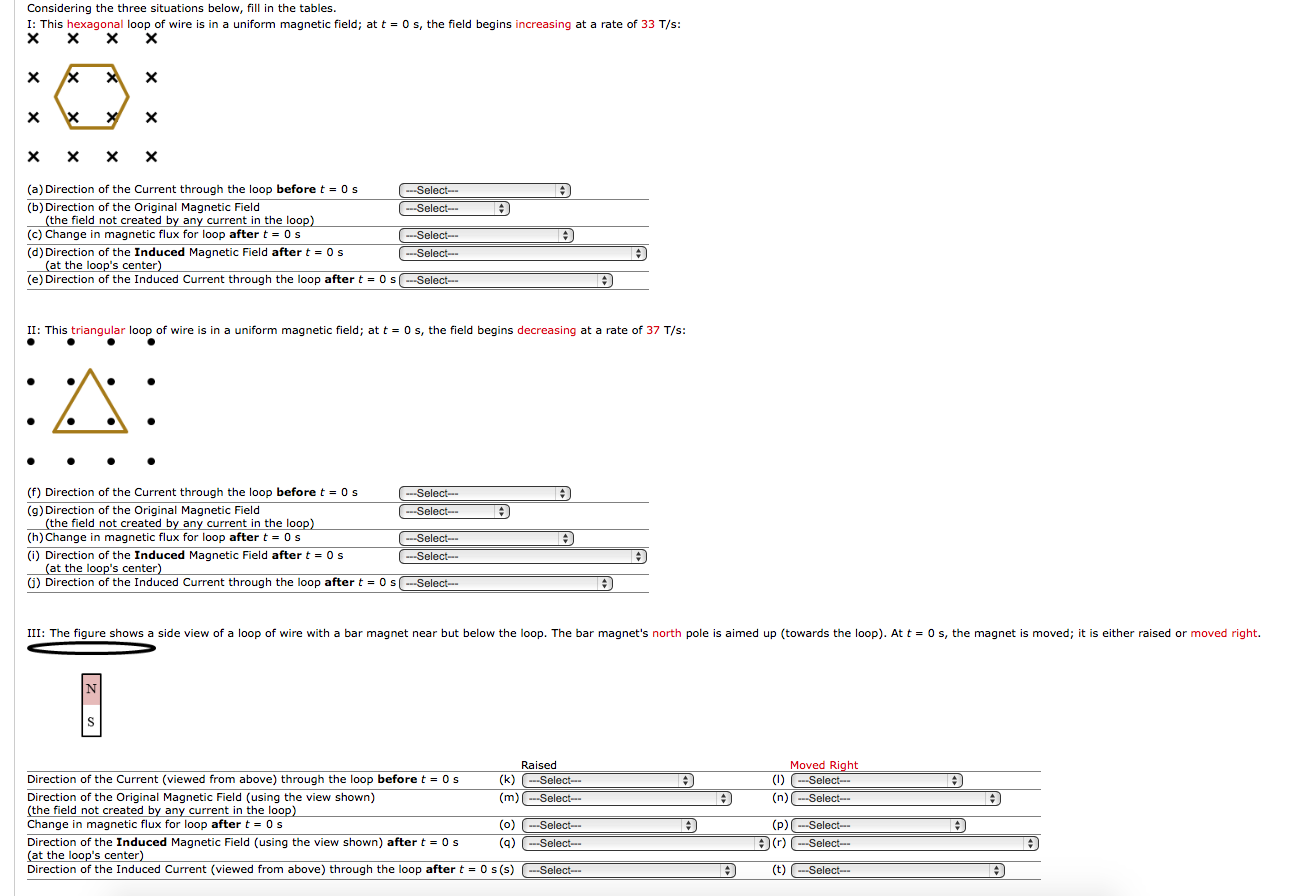For direction questions the choices are: into page, out of page, clockwise, counterclockwise, or no induced field. Letters (m), (n), (q) and (r) have choices: up, down, left, right, into page, out of page, or no induced field. The change in flux is either increasing, decreasing, or constant. Thank you for your help!

I.)

a.) Change in the magnetic flux causes induced current, not the magnetic field itself. So, before t = 0 , there is no current induced.

b.) Into the page. As indicated by the x marks.

c.) Increases as the magnetic field is also increasing continually

d.) Indued magnetic field always tries to oppose the change. So, Induced magnetic field points out of the page to oppose the increasing magnetic field going into the page.

e.) By using right-hand rule we can say that the induced current needs to be in the anti-clockwise direction to give an induced magnetic field out of the page.

II.)

f.) Change in the magnetic flux causes induced current, not the magnetic field itself. So, before t = 0 , there is no current induced.

g.) Out of the page. As indicated by the dots.

h.) Decreases as the magnetic field is also decreasing continually

i.) Induced magnetic field always tries to oppose the change. So, Induced magnetic field points out of the page to support the decreasing magnetic field coming out of the page.

j.) By using right-hand rule we can say that the induced current needs to be in the anti-clockwise direction to give an induced magnetic field out of the page.

III.)

k) & l) There is no current before t=0 because the magnetic field is not changing

m) & n.) Magnetic field lines come out of the north pole and go into the south pole of a magnet. So, the field lines point upwards.

o.) Increases because magnetic field lines get more intense as we get closer to the magnet.

p.) Decrease because the intensity of the magnetic field lines through the loop would decrease as we move the magnet towards the right.

q.) Down. As said before, the induced magnetic field always tries to oppose the change in the magnetic flux and in order to oppose the magnetic field increasing upwards, the induced magnetic field acts in the downward direction.

r.) Up. In order to support the decreasing magnetic field in the upward direction, the induced magnetic field also acts upwards.

s.) using the right-hand rule we get that the current when looked from above needs to in the clockwise direction to generate a magnetic field in the downward direction

t.) using the right-hand rule we get that the current when looked from above needs to in the anticlockwise direction to generate a magnetic field in the upward direction

#### Earn Coins

Coins can be redeemed for fabulous gifts.

Similar Homework Help Questions
• ### Hello, these two screen shots are one entire problem not two separate ones so PLEASE answer ALL of the parts to the ques...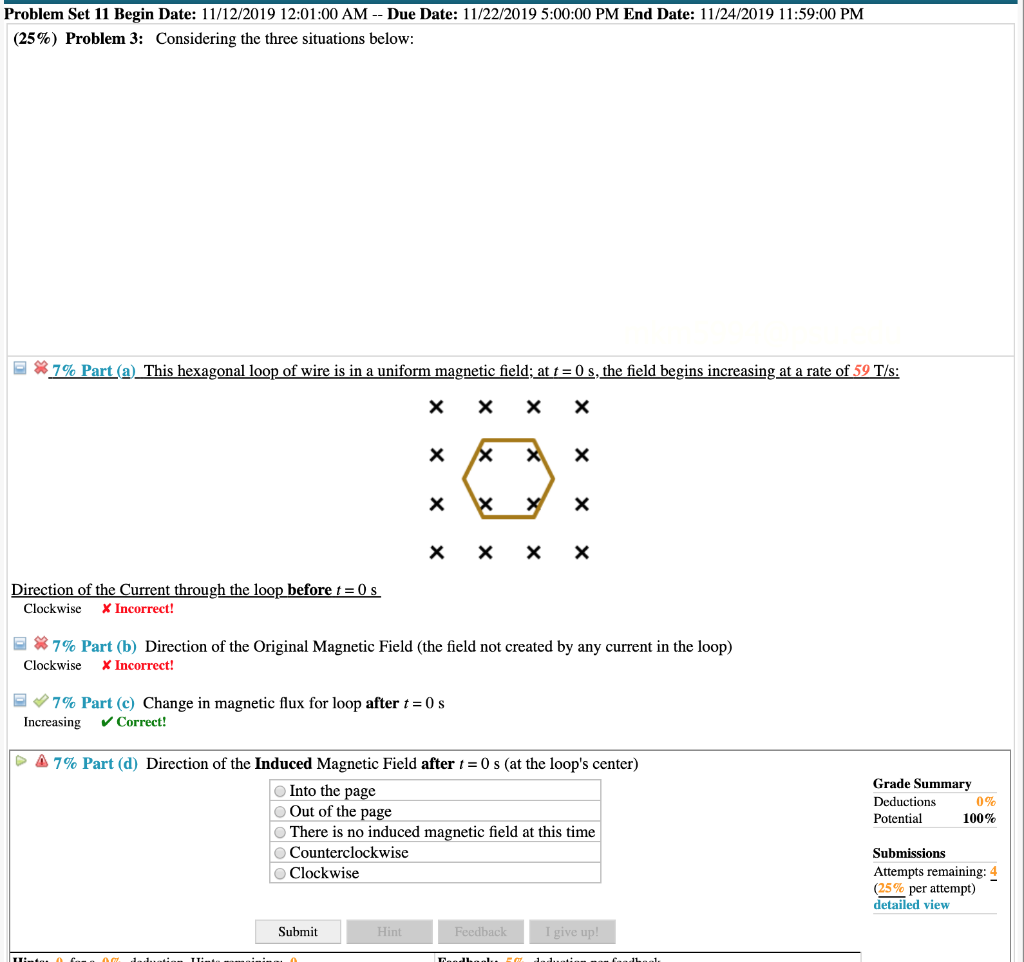Hello, these two screen shots are one entire problem not two separate ones so PLEASE answer ALL of the parts to the question! THANKS Problem Set 11 Begin Date: 11/12/2019 12:01:00 AM -- Due Date: 11/22/2019 5:00:00 PM End Date: 11/24/2019 11:59:00 PM (25%) Problem 3: Considering the three situations below: *7% Part (a) This hexagonal loop of wire is in a uniform magnetic field; at t = 0 s, the field begins increasing at a rate of 59 T/s:...

• ### (Mark two answers) As shown below, a current flows counter-clockwise around a wire loop. The direction...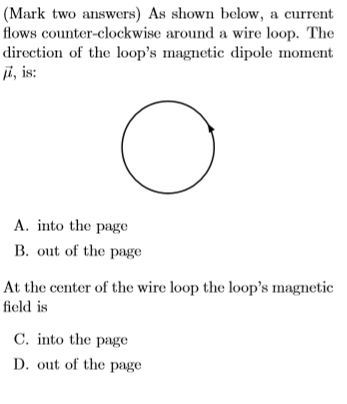(Mark two answers) As shown below, a current flows counter-clockwise around a wire loop. The direction of the loop's magnetic dipole moment , is: A. into the page B. out of the page At the center of the wire loop the loop's magnetic field is C. into the page D. out of the page

• ### ignore 2.21 The relationship you discovered is called Faraday's Law and is written as A Equation...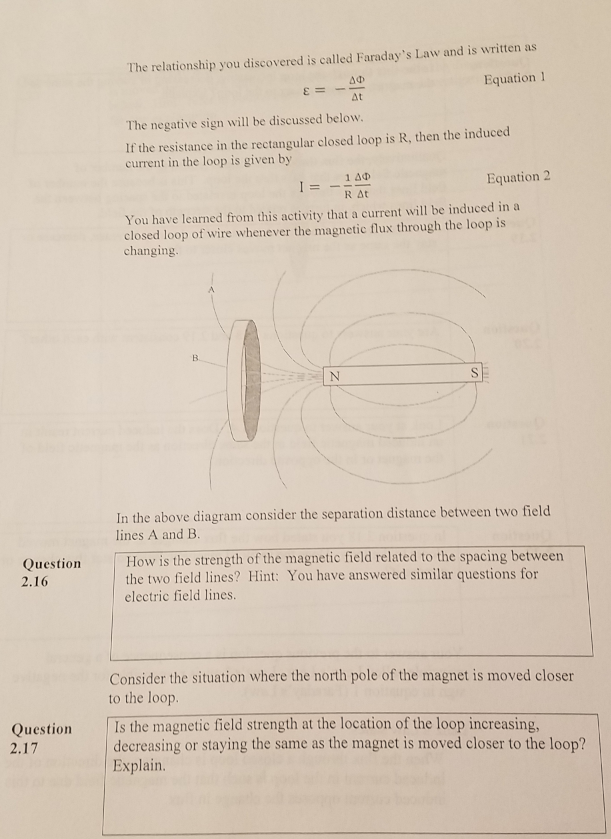ignore 2.21 The relationship you discovered is called Faraday's Law and is written as A Equation 1 E = At The negative sign will be discussed below. If the resistance in the rectangular closed loop is R, then the induced current in the loop is given by Equation 2 T = - 140 I RAT You have learned from this activity that a current will be induced in a closed loop of wire whenever the magnetic flux through the loop...

• ### Problem 7: For each situation shown below, indicate • the direction of the area vector you...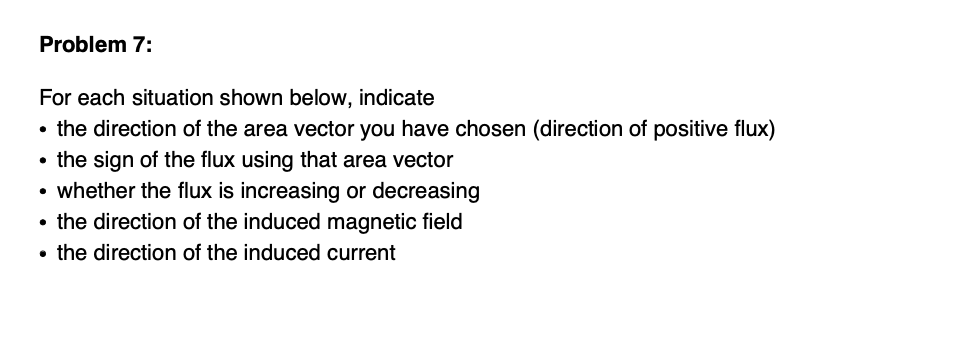Problem 7: For each situation shown below, indicate • the direction of the area vector you have chosen (direction of positive flux) the sign of the flux using that area vector • whether the flux is increasing or decreasing the direction of the induced magnetic field • the direction of the induced current . • the direction of the net force on the loop Loop of wire I - Increasing a) Loop near a wire with current increasing Loop of...

• ### Magnetic induction lab: 5 questions A vertical bar magnet is dropped through the center of a...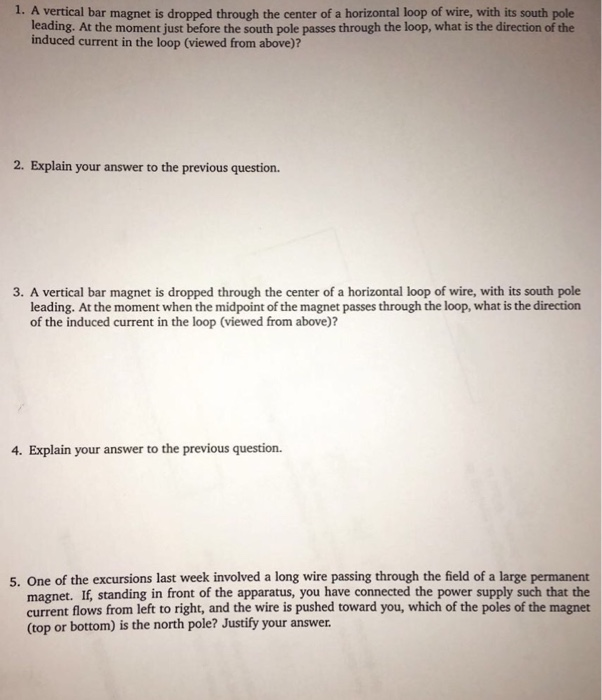Magnetic induction lab: 5 questions A vertical bar magnet is dropped through the center of a horizontal loop of wire, with its south pole leading. At the moment just before the south pole passes through the loop, what is the direction of the induced current in the loop (viewed from above)? 2. Explain your answer to the previous question. 3. A vertical bar magnet is dropped through the center of a horizontal loop of wire, with its south pole leading....

• ### Quiz 1: Consider a circular loop with a 2.50-cm radius in a constant magnetic field of...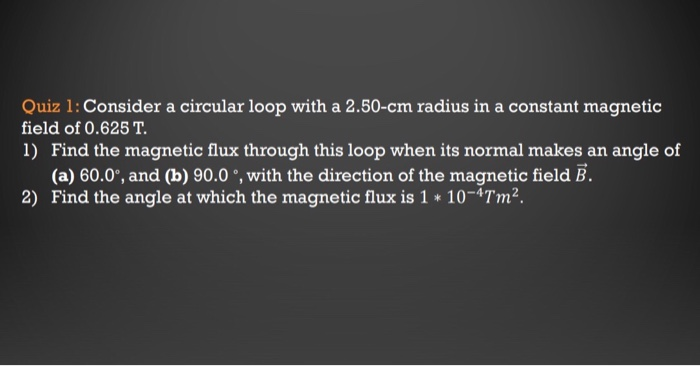Quiz 1: Consider a circular loop with a 2.50-cm radius in a constant magnetic field of 0.625 T. 1) Find the magnetic flux through this loop when its normal makes an angle of (a) 60.0°, and (b) 90.0°, with the direction of the magnetic field B. 2) Find the angle at which the magnetic flux is 1 * 10-*Tm. Quiz 2: A bar magnet is moved rapidly toward a 40-turn circular coil of wire. As the magnet moves, the average...

• ### What is the direction of the induced current in the loop?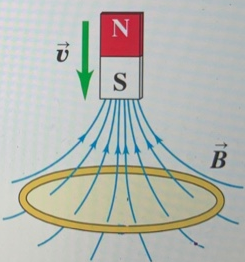What is the direction of the induced current in the loop? Please answer using Lenz's Law and explain the direction for each criterion.What is the direction of the induced current in the loop? A clockwiseB counterclockwise111C no current(a) Motion of magnet causes increasing downward flux through loop. How to use Lenz's law? (c) Motion of magnet causes decreasing downward flux through loop.

• ### 1) Which of Maxwell's equations below predicts an induced magnetic field due to changes in an...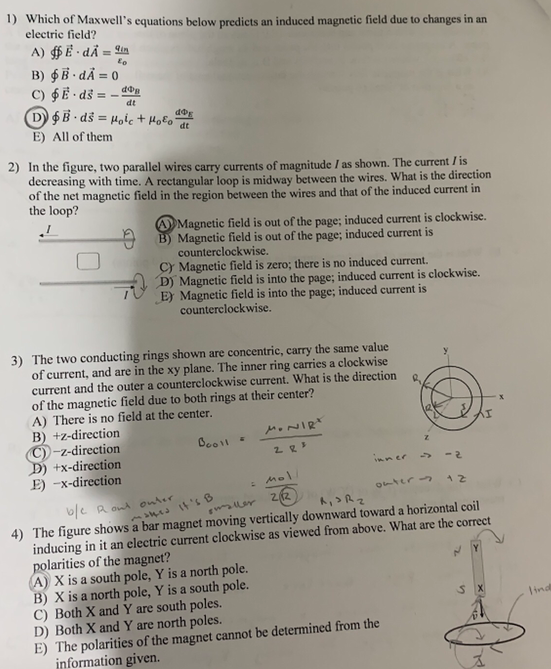1) Which of Maxwell's equations below predicts an induced magnetic field due to changes in an electric field? A) #Edi = 9 B) B. dA= 0 D\$B.ds = Hole + Hope E) All of them 2) In the figure, two parallel wires carry currents of magnitude / as shown. The current is decreasing with time. A rectangular loon is midway between the wires. What is the direction of the net magnetic field in the region between the wires and that...

• ### To practice Tactics Box 25.1Using Lenz's law. Lenz's law is a useful rule for determining...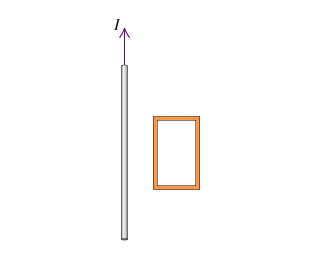To practice Tactics Box 25.1 Using Lenz's law. Lenz's law is a useful rule for determining the direction of the induced current in a loop. Specifically, it says that there is an induced current in a closed conducting loop if and only if the magnetic flux through the loop is changing. The direction of the induced current is such that the induced magnetic field opposes the change in the flux. The following Tactics Box summarizes the essential steps in using...

• ### only part C Bext 2. A single circular loop of wire is exposed to an external...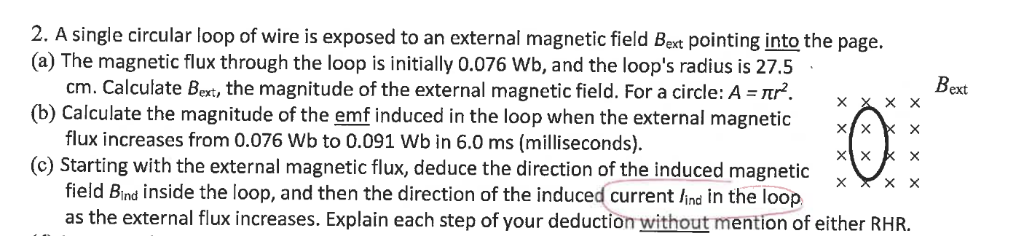only part C Bext 2. A single circular loop of wire is exposed to an external magnetic field Bext pointing into the page. (a) The magnetic flux through the loop is initially 0.076 Wb, and the loop's radius is 27.5 cm. Calculate Bext, the magnitude of the external magnetic field. For a circle: A = Ttr?. xxxx (b) Calculate the magnitude of the emf induced in the loop when the external magnetic flux increases from 0.076 Wb to 0.091 Wb...